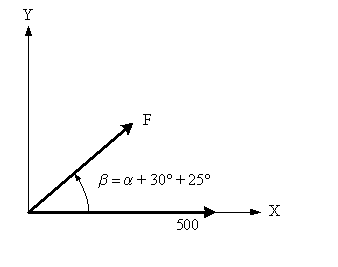# Equilibrium problem,

Lifprasir

## Homework Statement

http://puu.sh/4NWw9.PNG [Broken]

question 2.65**

## Homework Equations

I've tried using the law of sine and law of cosine method with the triangle for equilibrium and I've tried the 600^2 = Fx^2 + Fy^2

## The Attempt at a Solution

Here is what I did using the F^2 = Fx^2 + Fy^2

http://puu.sh/4NWDa.jpg [Broken]

after that

36027 = 2*272*(500*cos 30)*cos *beta - 2*272*(500*sin 30)*cos beta

36027/(2*272*500(cos beta (cos 30-sin 30))

I get beta is 68.78 , and since beta = alpha + 25, alpha = 43.78.

However, the answer in the book is 27.4 to 222.6. I was able to get 42.6 which is just 222.6 -180, using the triangle method (i really don't know what it's called, but it's when you put draw two forces together, and you can draw the resultant force and use trig laws) but got stuck figuring out the lowest angle.

http://puu.sh/4NXg2.jpg [Broken]

Here, I started off the same way as before by combining the two bottom vectors, and then I found the angle between the remaining two vectors is alpha + 55 then proceeded with a cos law, and found alpha to be 42.6 (now I am not sure if it's correct, since the answers on the back of the book specifically says 27.4 to 222.6, which is realistically impossible since the force can't go into the wall from the diagram).

Anyways, even from this method, I would not know how to solve for the lowest range of alpha, I can't set the resultant force to be 0, because there is no angle for R to be 0.

I've 2 days, a total of a good 5 hours trying to solve this, I would be blessed if someone could help me out :)

Last edited by a moderator:

Mentor
Sometimes a change of axes can make things dramatically easier. Align your positive X-axis through the 500N force. That eliminates dealing with any y-components for that vector. Your method of combining the two other forces into one with an angular offset is excellent. Add that to the mix. Then you get a situation that looks like this:Another hint: scale the problem so that the length of the vector F becomes 1. That is, divide both vectors by |F|. Note that angles are in no way affected by a change of scale.

#### Attachments

Lifprasir
mm, okay I followed your procedure, and I was able to get 27.47 as the angle for which gives 600N like this:

setting x = alpha + 55

sum of y-axis = sin x
sum of x-axis = cos x + 1.838
600/272 = 2.20

so, 2.20^2 = sin^2 x + cos^2 + 1.838^2 + 2*1.838*cos x
it simplifies to 0.131054 = cos (x)

and after substitution, i will find alpha is 27.47. I double checked this degree and was able to get 600N as the resultant force, which is great. Now getting the angle for lowest R,

R^2 - 4.378 = 3.676cos x, looking at this expression. I know that R can't be zero. Am I supposed to derive R = sqrt(4.378 + 3.676cos x), and set DR/Dx to zero and solve for x?? the derivative that wolframalpha gives me doesn't look too friendly. :S

Mentor
Too complicated!There are two angles that will satisfy cos(x) = <something>. What's the other one?

Lifprasir
Okay, so, I can do arccos (0.131054) = 82.47, but the other angle that would also satisfy this would be -82.47, or 277.53, now substracting 55, i would get 222.53 for alpha. This 222.53 would result in a resultant vector of 600N, but how do i know, in between 27.47 to 222.53, the resultant vector is under 600N? I mean , we only found the points where the resultant vector would 600N.

Mentor
Okay, so, I can do arccos (0.131054) = 82.47, but the other angle that would also satisfy this would be -82.47, or 277.53, now substracting 55, i would get 222.53 for alpha. This 222.53 would result in a resultant vector of 600N, but how do i know, in between 27.47 to 222.53, the resultant vector is under 600N? I mean , we only found the points where the resultant vector would 600N.

If a function f(x) has two zero crossings with no other crossings in between, there are only two possibilities for the sign of the values of f(x) between those crossings: either they're all positive or they're all negative. Test an angle in between your solution values to see which portion of the full circle encompasses your solution set.

Lifprasir
right! I remember that theorem from calculus I, thank you so much! I appreciate the help a lot. :)